# Data analysis - subtotals

## 1. Subtotals

One of the simplest ways to summarise worksheet data is to add subtotals.

Example: An analyst is interested in the sales data shown in figure 1, worksheet(1) named Sales Data 1.

This data set is constructed as follows:- row 1 is the header or labels row, and describes the fields in each column. This text data has some labels containing space characters. For example, worksheet column A is labeled Invoice No. The numbers 150100, 150101, 150102, ... are in the Invoice No fields. There are 11 fields in total. Each row in the body of the data is one unique record. Some blank or empty cells are OK, but there should be no empty rows or columns in the data set. When you click in a cell within the data, the data set becomes the Current Region.

## 2. Subtotals - manual

Reference: figure 1 Worksheet: Sales Data 1. Suppose that the Analyst is interested in the sales volume achieved by individual salespersons and intends to add subtotals to the data.

Subtotals can be added manually by:

1. Sorting the data set on the Salesperson column - see figure 2
2. To sort the data in ascending order, select a cell in the Salesperson column (field)
3. Then select the sequence Data > Sort & Filter > AZ↓ on the ribbon, or
4. The shortcut sequence Alt A SA
5. The data for Jellison ends at row 18
6. Insert a new row at row 19, then use the SUM function, or the SUBTOTAL function to summarise the data
7. Repeat this process for the other salespersons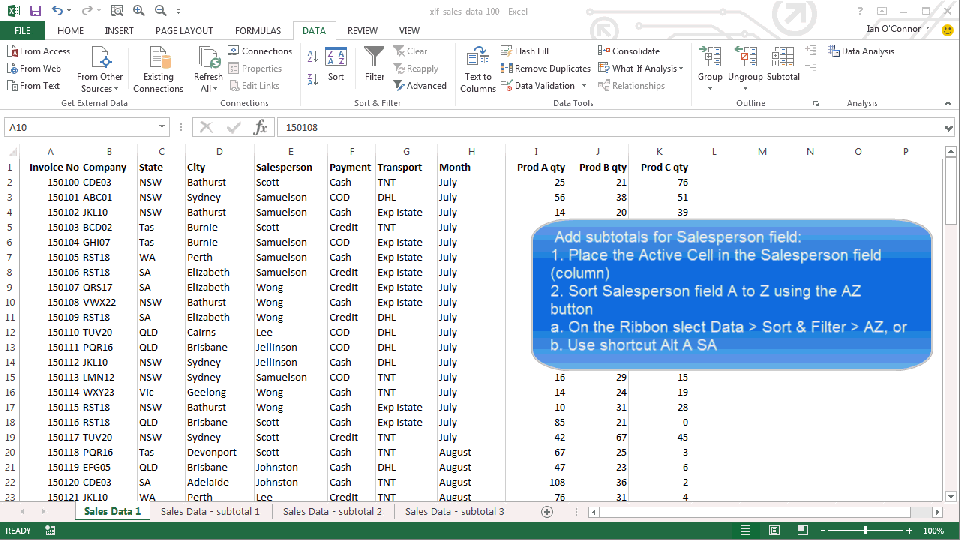Fig 2: Sorting Current Region - xlfAnimatedPreview - select a cell in the sort column,then the AZ↓ sort button the ribbon

An easier method is to use the Excel subtotal command. Use of the command is shown in the next three subsections.

## 3. Subtotal command

### 3.1 Subtotals - single

Using the same data sort from figure 2, the data is repeated in worksheet(2) named Sales data - subtotal 1.

Subtotals with the subtotal command:

1. Click on a cell in the data set
2. Then select the sequence Data > Outline > Subtotal on the ribbon, or
3. The shortcut sequence Alt A B
4. This displays the Subtotal dialog box- see figure 3
5. Figure 3 - the Subtotal dialog box options - At each change in: select Salesperson from the drop down list, Use function: Subtotal, and Add subtotal to: the three quantity columns
6. Click OK - to display the list in figure 4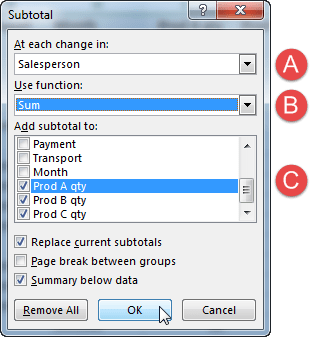Fig 3: Subtotal dialog box - Ⓐ At each change in: Label drop down list, Ⓑ Use function: Subtotal, and Ⓒ Add subtotal to: Label drop down list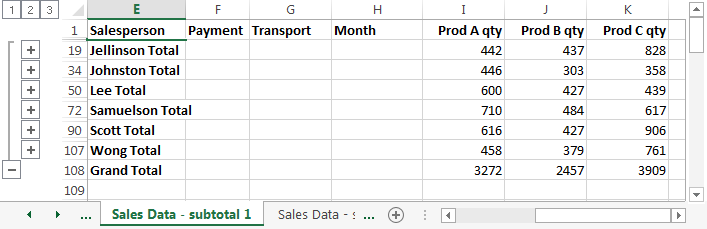Fig 4: Subtotal - Salesperson field, Sum function subtotal

The subtotal command adds groups to the data set, with expand and collapse items in the subtotal panel to the left of the row header item. See figure 4.

• The expand / contract feature is used by clicking on a level in the $\boxed{1}$, $\boxed{2}$, $\boxed{3}$ sequence or a group a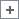,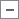item
• $\boxed{1}$ - Grand Total
• $\boxed{2}$ - Salesperson Total
• $\boxed{3}$ - full detail, or click a level 2to expand individual Salesperson detail
• Clickorto expand or contract selected group
• To hide or view the subtotal panel press Ctrl+8

### 3.2 Subtotals - multi

Worksheet(3) named Sales data - subtotal 2.

1. To add an Average subtotal to the existing Sum subtotal, Use function: Average, and
2. untick Replace current subtotals (figure 5) to prevent the Sum being overwritten
3. The result is shown in figure 6 - with 4 levels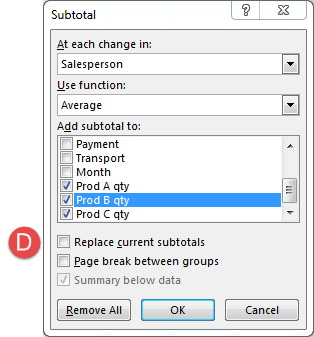Fig 5: Subtotal dialog box - Ⓓ untick Replace current subtotalsFig 6: Subtotal - Salesperson field, Sum and Average function multi subtotal

### 3.3 Subtotals - nested

Worksheet(4): Sales Data - subtotal 3

The AZ↓ sort command can only sort on one field. To setup a nested subtotal, two or more fields need to be sorted. This requires the multi level features of the Sort dialog box - figure 7.

To set up a Salesperson subtotal, with a Transport nested subtotal

1. Using the Sort dialog box, Sort by: Salesperson, Then by: Transport
2. Set the subtotal for Salesperson - figure 3, then
3. Set the subtotal for Transport - untick Replace current subtotals
4. The result is shown in figure 8 - with 4 levels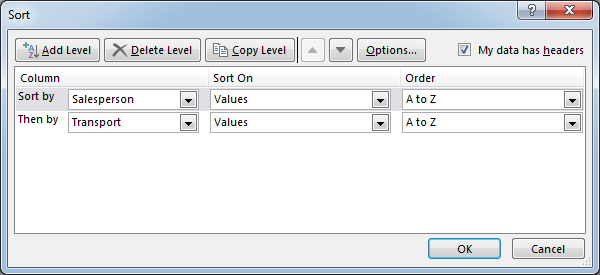Fig 7: Sort dialog box - with settings for level 1: Salesperson, and level 2: Transport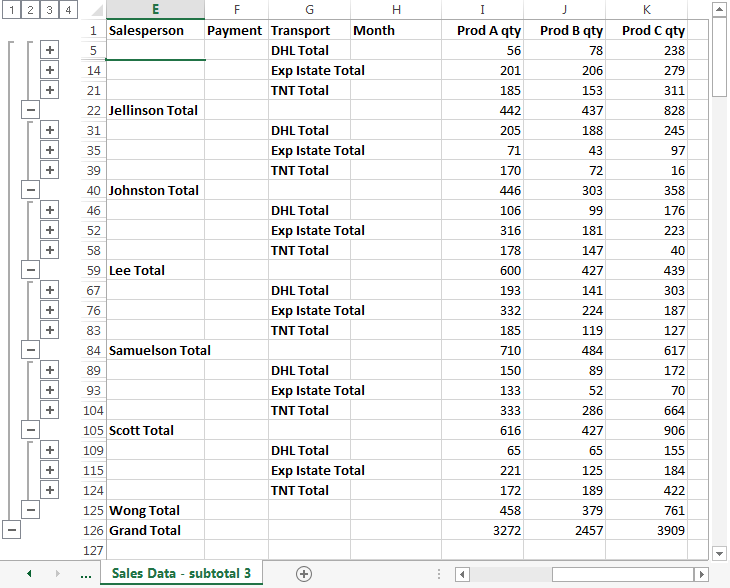Fig 8: Subtotal - Salesperson field, Sum function subtotal, nested subtotal Transport field, Sum function

### 3.4 Format subtotals

Any cell formats applied to subtotals, are removed when the subtotals are cleared.

To clear subtotals, click the Remove All button in the Subtotal dialog box.

### 3.5 Copy visible subtotal cells

To copy the visible cells (visible subtotal data)

1. Select the data to be copied - the Source
2. Press F5 to display the Go To Special dialog box
3. Select Visible cells only
4. Click OK
5. Press Ctrl+C to copy the source to the clipboard
6. Select the cell for the target, then press Ctrl+V to Paste.

Note: steps 2 to 4 can be replaced by the Alt+; keyboard shortcut (see EXCEL: useful keyboard shortcuts - pdf)

## 4. Subtotal function

The subtotal procedure used the Excel subtotal function

Syntax: SUBTOTAL(function_num,ref1,[ref2],...)

Function_num
(includes hidden values)
Function_num
(excludes hidden values)
Function
1101AVERAGE
2102COUNT
3103COUNTA
4104MAX
5105MIN
6106PRODUCT
7107STDEV.S (replaced STDEV in xl2010)
8108STDEV.P (replaced STDEVP in xl2010)
9109SUM
10110VAR.S (replaced VAR in xl2010)
11111VAR.P (replaced VARP in xl2010)

### 4.1 Using SUBTOTAL for summary statistics

You can use the SUBTOTAL function to indirectly link cell labels to the summary statistic procedure - figure 9.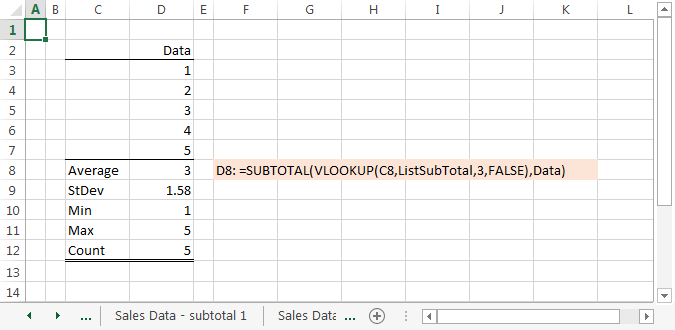Fig 9: Subtotal function as dynamic link to label - Subtotal with the function_num argument linked to a function argument array named ListSubTotal

The example in figure 9 is available on the Summary Stats worksheet - Excel Web App #1

The ListSubTotal array has the values  ={"average",101,1;"count",102,2;"counta",103,3;"max",104,4; "min",105,5;"product",106,6;"stdev",107,7;"stdevp",108,8;"sum",109,9;"var",110,10;"varp",111,11}